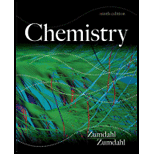# Consider the reaction 3A+B+C → D+E where the rate law is defined as − Δ [ A ] Δ t = k [ A ] 2 [ B ] [ C ] An experiment is carried out where [B] 0 = [C] 0 = 1.00 M and [A] 0 = 1.00 × 10 −4 M. a. If after 3.00 min, [A] = 3.26 × 10 −5 M, calculate the value of k. b. Calculate the half-life for this experiment. c. Calculate the concentration of B and the concentration of A after 10.0 min.### Chemistry

9th Edition
Steven S. Zumdahl
Publisher: Cengage Learning
ISBN: 9781133611097### Chemistry

9th Edition
Steven S. Zumdahl
Publisher: Cengage Learning
ISBN: 9781133611097

#### Solutions

Chapter
Section
Chapter 12, Problem 93AE
Textbook Problem

## Expert Solution

### Want to see the full answer?

Check out a sample textbook solution.See solution

### Want to see this answer and more?

Experts are waiting 24/7 to provide step-by-step solutions in as fast as 30 minutes!*

See Solution

*Response times vary by subject and question complexity. Median response time is 34 minutes and may be longer for new subjects.